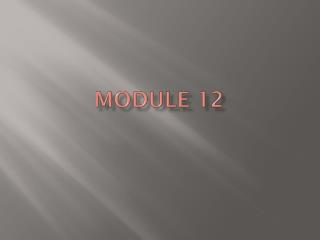DownloadDownload PresentationModule 12

# Module 12

Télécharger la présentation## Module 12

- - - - - - - - - - - - - - - - - - - - - - - - - - - E N D - - - - - - - - - - - - - - - - - - - - - - - - - - -
##### Presentation Transcript

1. Module 12

2. Objective: 1. After completing act. 1 2. Students will: • -apply definitions, axioms, & theorems • -investigate SSS & SAS congruence of tri.s • -identify included sides & angles • -write congruence statements 3. with 85% accuracy

3. Introduction to Mod. 12 • Please sit in pairs. Make sure to pair up with someone you can work with… • You will need 2 index cards, 4 pieces of tape, & 1 pair of scissors, a pencil, & your notebook. • As a class, we will read page 324 • In your pairs please complete the Exploration page 324-325 parts a-e

4. Activity 1 Note: Congruent Shapes: have equal corresponding sides, equal corresponding angles, the shapes are the same…

5. Lets review symbols in Geometry:

6. Exploration Activity 1 • As a class, we will read page 326 • With your partner complete, Exploration 1 on page 327 • Be ready to discuss the questions under Discussion 1 page 327-328 a-d

7. Angle-Angle-Angle (AAA) Property: • If the angles of one triangle are congruent to the angles of another triangle, then the triangle are similar. • Describe the characteristics of similar triangles • Can draw an example?

8. Included Angles: • Note on board. • With your partner, try Exploration 2 and be ready to discuss the questions under Discussion 2.

9. Conjecture: • Your opinion or theory about something without sufficient evidence or proof that it is true.

10. Class work: • With your partner(s): Complete Exploration 2 on page 328-329 parts a-l • Be ready with your answers to the Discussion 2 Questions regarding your exploration on page 329-330

11. Conditional Statement in “if-then” form : • A conditional statement consists of 2 parts: the hypothesis & the conclusion. • The hypothesis is the “if” part. • The conclusion is the “then” part. • For example: “If an animal is a German shepherd, then the animal is a dog.” • Identify the hypothesis: • If an animal is a German shepherd • Identify the conclusion: • then the animal is a dog

12. Conditional Statement: • If a triangle has a right angle, then the triangle is a right triangle. • What is the hypothesis? • What is the conclusion?

13. Side-Angle-Side (SAS) axiom: • If two sides and an included angle of one triangle are congruent to two sides and an included angle of another triangle, then the triangles are congruent. (Sketch on the board ) • Why do you think this is referred to the SAS axiom? What does axiom mean?

14. Side-Side-Side (SSS) Axiom: • If three sides of a triangle are congruent to three sides of another triangle, then the triangles are congruent. • (sketch on the board)

15. Vertical Angles: Vertical Angles are angles that are opposite from each other when two lines intersect. For example, in the sketch above angle DAC and BAE are vertical angles. Can you name another pair?

16. Vertical Angles Property: • Use a ruler & sketch two lines that intersect each other. • Identify a pair of vertical angles, label one angle as angle 1 and the other as angle 2. (Mrs. Ortiz will give an example on the board) Use a protractor and measure the two angles. What do you notice?

17. Vertical Angles Property: • Vertical angles are always congruent. • Use the sketch below and identify all the pairs of vertical angles:

18. Try the following questions: • Page 328 part e (the one above Exploration 2) • Page 330 part g

19. Your class work & Homework: • CW: page 333-334 Assignment #1.1, 1.3a-d, 1.5 • HW: page 332 warm-up #1-2

20. Objective: 1. After completing act. 2 2. Students will: • -apply definitions, axioms, & theorems • -write “if-then” conditional statements • -use direct proof as to establish theorems • -examine & write proofs of the Pythagorean Thm. 3. w/ 85% accuracy

21. Objective: 1. After completing act. 3 2. Students will: • -apply definitions, axioms, & theorems • write “if-then” conditional statements • -use direct proof as to establish theorems 3. With 85% accuracy.

22. Objective: 1. After completing act. 4 2. Students will: • -apply definitions, axioms, & theorems • -investigate & prove sine properties of quadrilaterals 3. With 90% accuracy